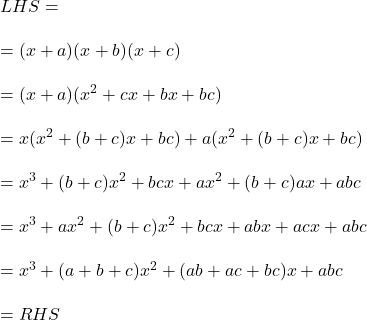## Verify that (x + a)(x+b)(x+c) is factor of f(x)= x³ +(a+b+c)x²+(ab + ac + bc)x + abc.​

Question

Verify that (x + a)(x+b)(x+c) is factor of f(x)= x³ +(a+b+c)x²+(ab + ac + bc)x + abc.​

in progress 0
4 weeks 2021-08-18T10:56:01+00:00 2 Answers 1 views 0

Step-by-step explanation: